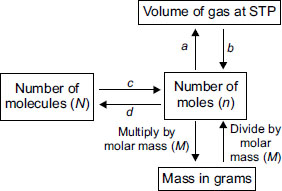# User Forum

Subject :NSO    Class : Class 9

Observe the given flow chart and answer the following questions. What are a and b?A a = multiply by 22.4 L, b = multiply by 22.4 L
B a = multiply by 22.4 L, b = divide by 22.4 L
C a = divide by 22.4 L, b = divide 22.4 L
D a = divide by 22.4 L, b = multiply by 22.4 L